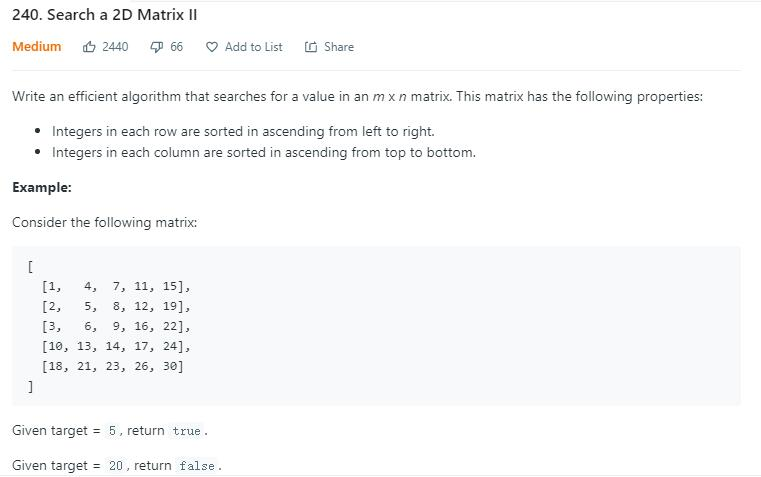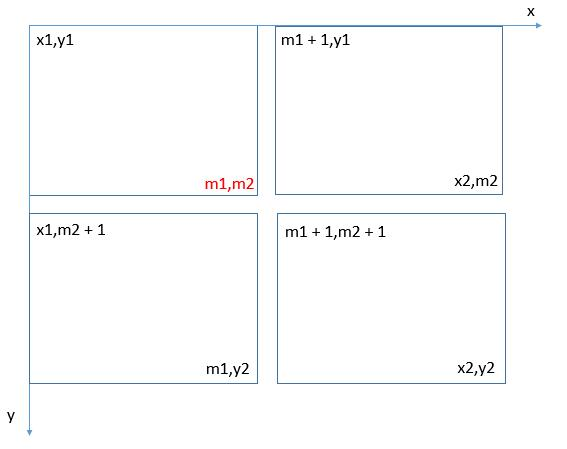# 题目描述（中等难度）# 解法一

public boolean searchMatrix(int[][] matrix, int target) {
if (matrix.length == 0 || matrix.length == 0) {
return false;
}
for (int i = 0; i < matrix.length; i++) {
if (matrix[i] > target) {
break;
}
if(matrix[i][matrix[i].length - 1] < target){
continue;
}
int col = binarySearch(matrix[i], target);
if (col != -1) {
return true;
}
}
return false;
}

//二分查找
private int binarySearch(int[] nums, int target) {
int start = 0;
int end = nums.length - 1;
while (start <= end) {
int mid = (start + end) >>> 1;
if (nums[mid] == target) {
return mid;
} else if (nums[mid] < target) {
start = mid + 1;
} else {
end = mid - 1;
}
}
return -1;
}


# 解法二

• 如果 target 的值大于当前值，那么就向下走。
• 如果 target 的值小于当前值，那么就向左走。
• 如果相等的话，直接返回 true

[1,   4,  7, 11, 15],
[2,   5,  8, 12, 19],
[3,   6,  9, 16, 22],
[10, 13, 14, 17, 24],
[18, 21, 23, 26, 30]

target < 15, 去掉当前列, cur = 11
[1,   4,  7, 11],
[2,   5,  8, 12],
[3,   6,  9, 16],
[10, 13, 14, 17],
[18, 21, 23, 26]

target < 11, 去掉当前列, cur = 7
[1,   4,  7],
[2,   5,  8],
[3,   6,  9],
[10, 13, 14],
[18, 21, 23]

target > 7, 去掉当前行, cur = 8
[2,   5,  8],
[3,   6,  9],
[10, 13, 14],
[18, 21, 23]

target > 8, 去掉当前行, cur = 9, 遍历结束
[3,   6,  9],
[10, 13, 14],
[18, 21, 23]


public boolean searchMatrix(int[][] matrix, int target) {
if (matrix.length == 0 || matrix.length == 0) {
return false;
}
int row = 0;
int col = matrix.length - 1;
while (row < matrix.length && col >= 0) {
if (target > matrix[row][col]) {
row++;
} else if (target < matrix[row][col]) {
col--;
} else {
return true;
}
}
return false;
}


# 解法三

如下图，中心位置是 9
[1,   4,  7, 11, 15],
[2,   5,  8, 12, 19],
[3,   6, /9/,16, 22],
[10, 13, 14, 17, 24],
[18, 21, 23, 26, 30]

[1,   4,  7   [11, 15
2,   5,  8    12, 19
3,   6, /9/]  16, 22]

[10, 13, 14   [17, 24
[18, 21, 23]   26, 30]public boolean searchMatrix(int[][] matrix, int target) {
if (matrix.length == 0 || matrix.length == 0) {
return false;
}
return searchMatrixHelper(matrix, 0, 0, matrix.length - 1, matrix.length - 1, matrix.length - 1, matrix.length - 1, target);
}

private boolean searchMatrixHelper(int[][] matrix, int x1, int y1, int x2, int y2, int xMax, int yMax, int target) {
//只需要判断左上角坐标即可
if (x1 > xMax || y1 > yMax) {
return false;
}

//x 轴代表的是列，y 轴代表的是行
if(x1 == x2 && y1 == y2){
return matrix[y1][x1] == target;
}
int m1 = (x1 + x2) >>> 1;
int m2 = (y1 + y2) >>> 1;
if (matrix[m2][m1] == target) {
return true;
}
if (matrix[m2][m1] < target) {
// 右上矩阵
return searchMatrixHelper(matrix, m1 + 1, y1, x2, m2, x2, y2, target) ||
// 左下矩阵
searchMatrixHelper(matrix, x1, m2 + 1, m1, y2, x2, y2, target) ||
// 右下矩阵
searchMatrixHelper(matrix, m1 + 1, m2 + 1, x2, y2, x2, y2, target);

} else {
// 右上矩阵
return searchMatrixHelper(matrix, m1 + 1, y1, x2, m2, x2, y2, target) ||
// 左下矩阵
searchMatrixHelper(matrix, x1, m2 + 1, m1, y2, x2, y2, target) ||
// 左上矩阵
searchMatrixHelper(matrix, x1, y1, m1, m2, x2, y2, target);
}
}